Author: Oscar Cronquist Article last updated on April 19, 2018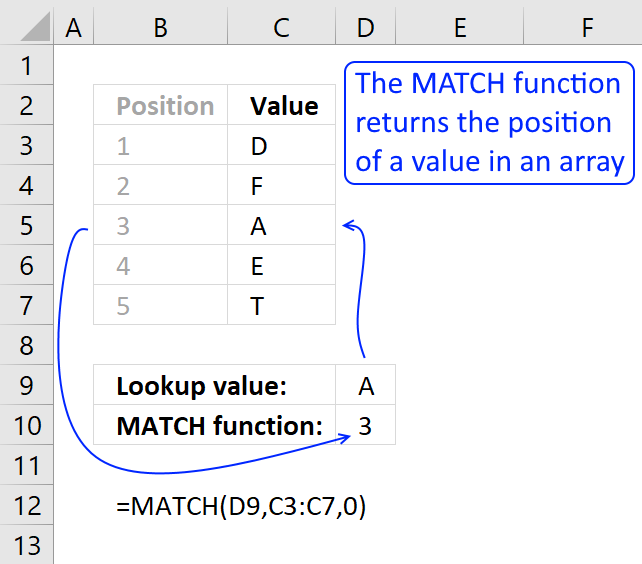Returns the relative position of an item in an array that matches a specified value in a specific order.

The formula in cell D10 searches for value "A" in cell range C3:C7 and finds an exact match in cell C5. The relative position of C5 in cell range C3:C7 is 3, shown in column B.

The formula returns 3 in cell D10, remember to use 0 (zero) in the third argument if you want to find an exact match.

Excel Function Syntax

MATCH(lookup_value, lookup_array, [match_type])

Arguments

 lookup_value Required. Is the value you use to find the value you want in the array, a number, text or logical value, or a reference to one of these. lookup_array Required. Is a contiguous range of cells containing possible lookup values, an array of values, or a reference to an array. [match_type] Optional. How to match, a number -1,0,1. If omitted number 1 is used.

match_type

 -1 Finds the largest value that is less than or equal to lookup_value. Lookup_array must be placed in ascending order 0 Finds the value that is exactly equal to lookup_value. Lookup_array can be in any order 1 Finds the smallest value that is greater than or equal to lookup_value. Lookup_array must be placed in descending order

• The lookup_array must be  a one dimensional horizontal or vertical cell range
• The lookup_value can also be an array, one or two dimensional. (array formula)
• The lookup_array can be one value. (array formula)
• You can use other excel functions in the lookup_value argument.
• You can use other excel functions in the lookup_array argument. As long as they return a one-dimensional array.
• I use match_type = 0 almost all the time.

Example 1 - Lookup_array in ascending order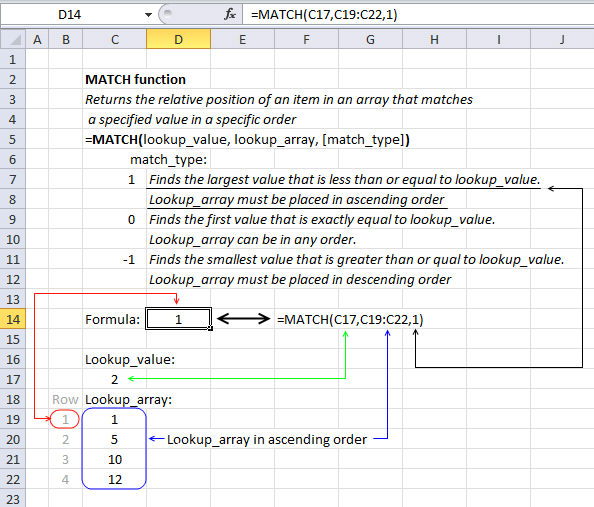=MATCH(C17, C19:C21,1)

becomes

MATCH(2, {1; 5; 10; 12},1)

Match_type 1 - Find the largest value {1; 5; 10; 12} that is less or equal to lookup_value (2)

MATCH(2, {1; 5; 10; 12},1)

The relative position of number 1 in array {1; 5; 10; 12} is 1. 1 is returned in cell D14.

Example 2 - Lookup_array in any order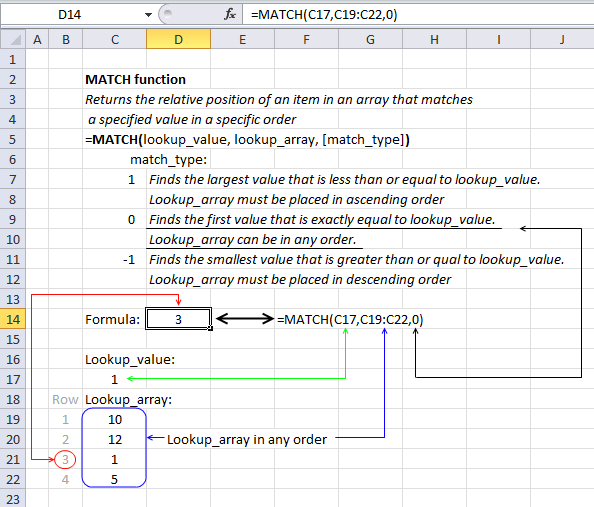=MATCH(C17, C19:C21,0)

becomes

MATCH(1, {10; 12; 1; 5},0)

Match_type 1 - Find the first value {10; 12; 1; 5} that is exactly equal to lookup_value (1)

MATCH(1, {10; 12; 1; 5},0)

The relative position of number 1 in array {10; 12; 1; 5} is 3. 3 is returned in cell D14.

Example 3 - Lookup_array in descending order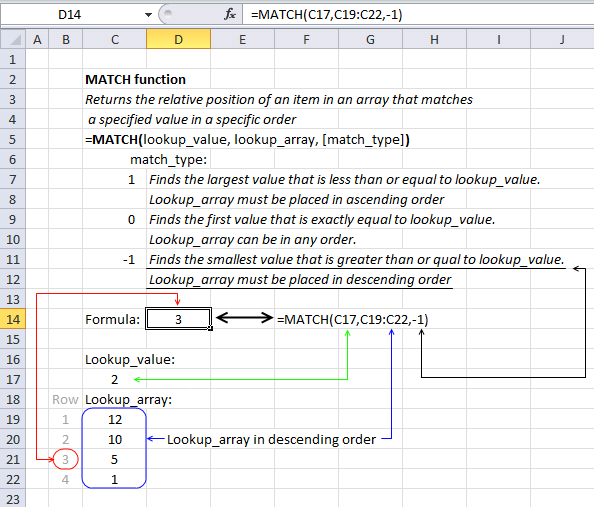=MATCH(C17, C19:C21,-1)

becomes

MATCH(2, {12; 10; 5; 1},-1)

Match_type 1 - Find the smallest value {12; 10; 5; 1} that is greater than or equal to lookup_value (2)

MATCH(2, {12; 10; 5; 1},-1)

The relative position of number 5 in array {12; 10; 5; 1} is 3. 3 is returned in cel D14.

Example 4 - Array formula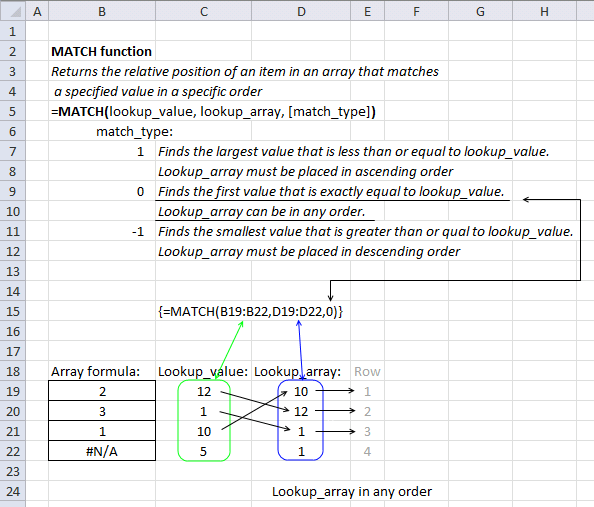=MATCH(C19:C22, D19:D22,0)

becomes

MATCH({12;1;10;5}, {10; 12; 1; 1},0)

Match_type 1 - Find the first value {10; 12; 1; 1} that is exactly equal to lookup_value {12;1;10;5}

Lookup value 12 is the second value in the lookup_array {10; 12; 1; 1}.

Lookup value 1 is the third value in the lookup_array {10; 12; 1; 1}.

Lookup value 10 is the first value in the lookup_array {10; 12; 1; 1}.

Lookup value 5 is not found in the lookup_array {10; 12; 1; 1}.

{2; 3; 1; #N/A} is returned in cell range B19:B22.

fghdfn

VBA Example

The following macro uses the MATCH function to find the position of the lookup value in cell range C5:C8. It then displays a message box containing that position.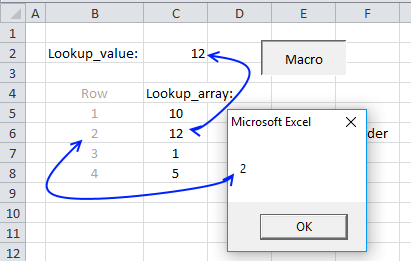VBA code

```Sub VBA_MATCH()
MsgBox Application.WorksheetFunction.Match(Range("C2"), Range("C5:C8"), 0)
End Sub
```